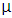# An amplifier with resistive negative feedback has 2 left half plane poles in its open-loop transfer function. The amplifier

1.   Will always be unstable at high frequencies

2.  Will be stable for all frequencies

3.  May be unstable, depending on feedback factor

4.  Will oscillate at low frequencies

4

Will be stable for all frequencies

Explanation :
No Explanation available for this question

# The circuit shown is

1.  Fast precision rectifier

2.  Saw tooth wave generator

3.  Special bridge rectifier

4.  Sample and hold

4

Fast precision rectifier

Explanation :
No Explanation available for this question

# A coil with Q=10 is put in series with capacitor C1=10F and the combination is found to draw maximum current when a sinusoidal voltage of frequency 50Hz is applied. A second C2 is now connected in parallel with the circuit. What should be the capacitance of C2 for the combined circuit to act purely as a resistance for a sinusoidal excitation at f=100Hz

1.  5.2F

2.  2.6F

3.  3.4F

4.  4.8F

4

3.4F

Explanation :
No Explanation available for this question

# An amplifier is assumed to have a signal pole high frequency transfer function. Rise time of its output response to a step function input is 33ns. Upper 3dB frequency for sinusoidal input will be

1.  5Hz

2.  10Hz

3.  20Hz

4.  30Hz

4

30Hz

Explanation :
No Explanation available for this question

# An npn transistor with C=0.3pF has an unity gain cutoff frequency ft=400 MHz at bias current IC=1mA. The value of Cπ is

1.  0.051pF

2.  0.03pF

3.  50pF

4.  96pf

4

0.051pF

Explanation :
No Explanation available for this question

# Statement I: In a differential amplifier, CMRR can be improved by using an increased emitter resistance Statement II: From a measurement of rise time of output pulse of an amplifier whose input is a small amplitude square wave, we can estimate the slew rate.

1.  Both are true

2.  Both are false

3.  Only I is false

4.  Only I is true

4

Only I is true

Explanation :
No Explanation available for this question

# Given a system represented by equations and y = [1 0]x The equivalent transfer function representation G(s) of the system is

1.

2.

3.

4.

4Explanation :
No Explanation available for this question

# Consider the following properties attributed to state model of a system 1. State model is unique 2. State model can be derived from the system transfer function 3. State model can be derived from time variant systems Of these statements:

1.   1, 2 and 3 are correct

2.  1 and 2 are correct

3.  2 and 3 are correct

4.  1 and 3 are correct

4

2 and 3 are correct

Explanation :
No Explanation available for this question

# A second order system is given by d2y/dt2 + 12 dy/dt + 100 y = 0. The damped natural frequency in rad/sec is

1.  100

2.  10

3.  √44

4.   8

4

8

Explanation :
No Explanation available for this question

# The transfer function C(s)/R(s) of the system, whose block diagram is given below is

1.

2.

3.

4.

4